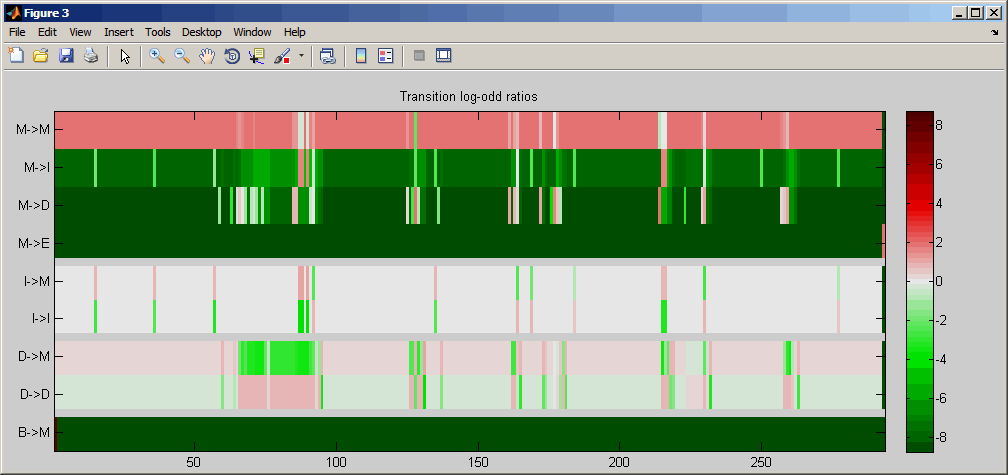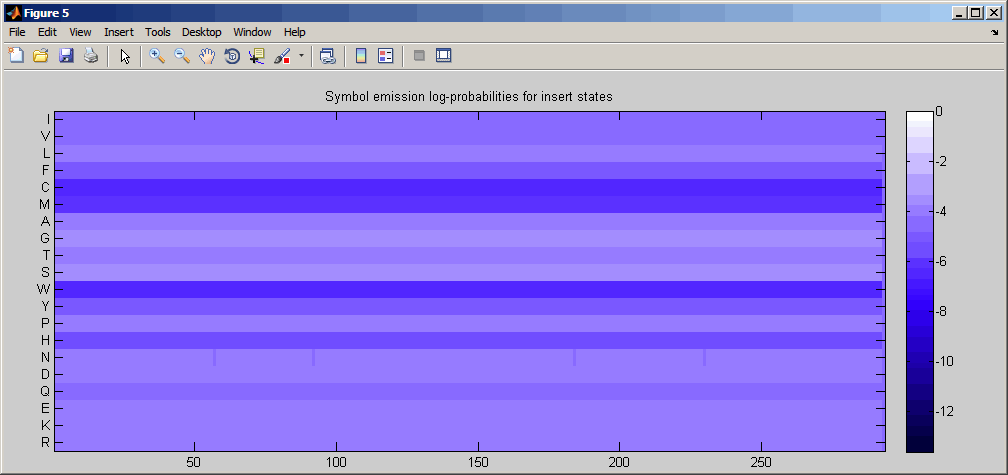# showhmmprof

Plot hidden Markov model (HMM) profile

## Syntax

```showhmmprof(Model) showhmmprof(Model, ...'Scale', ScaleValue, ...) showhmmprof(Model, ...'Order', OrderValue, ...) ```

## Arguments

 `Model` Hidden Markov model created by the function `gethmmprof` or `pfamhmmread`. `ScaleValue` Property to select a probability scale. Enter one of the following values:`'logprob'` — Log probabilities`'prob'` — Probabilities`'logodds'` — Log-odd ratios `OrderValue` Property to specify the order of the amino acid alphabet. Enter a character vector or string with the 20 standard amino acids characters ```A R N D C Q E G H I L K M F P S T W Y V```. The ambiguous characters ```B Z X``` are not allowed.

## Description

`showhmmprof(Model)` plots a profile hidden Markov model described by the structure `Model`.

```showhmmprof(..., 'PropertyName', PropertyValue, ...)``` calls `showhmmprof` with optional properties that use property name/property value pairs. You can specify one or more properties in any order. Each `PropertyName` must be enclosed in single quotation marks and is case insensitive. These property name/property value pairs are as follows:

``` showhmmprof(Model, ...'Scale', ScaleValue, ...) ```specifies the scale to use. If log probabilities (`ScaleValue='logprob'`), probabilities (`ScaleValue='prob'`), or log-odd ratios (`ScaleValue='logodds'`). To compute the log-odd ratios, the null model probabilities are used for symbol emission and equally distributed transitions are used for the null transition probabilities. The default `ScaleValue` is `'logprob'`.

```showhmmprof(Model, ...'Order', OrderValue, ...)``` specifies the order in which the symbols are arranged along the vertical axis. This option allows you reorder the alphabet and group the symbols according to their properties.

## Examples

`model = pfamhmmread('pf00002.ls');`
2. Plot the profile.

`showhmmprof(model, 'Scale', 'logodds')`3. Order the alphabet by hydrophobicity.

`hydrophobic = 'IVLFCMAGTSWYPHNDQEKR';`
4. Plot the profile.

`showhmmprof(model, 'Order', hydrophobic)`